• ### maths regarding conversion from metro speed to pitch

my deficiency in some higher math principles has me stumped in PD sometimes and im guessing this is a another example.

i have a 12 point array as a wavetable in a tutorial i had seen and the speed of the metro into tabread to vline~ determines the pitch...

using sigmund~ i determined the following relationships between the metro speed and the pitch output...
.01 = 95.96, .1 = 80.05, .2 = 68.05, .25 = 64.19, .3 = 61.03, .4 = 56.05, .5 = 52.19

here is where i am stumped... how do i make a conversion formula for this?

any insight would help!

thanks!

• Posts 20 | Views 837
• @murkrellr Well, for what it's worth, here are my whiskey-soaked observations on this very dead USA good Friday evening:

As others have been telling you, metro's speed is set by specifying the period (i.e. time between bangs, in mS). So if you think of each bang as a new "cycle", that's mS/cycle. But frequency is often specified in cycles per second, i.e. cycles/S. So at the very least there's a factor of 1000 in there somewhere, and the fraction is flipped upside down, i.e. raised to the -1 power.

OK, let's ignore the factor of a 1000 for the time being because I have no idea what your patch is doing and I can see that between 0.1 and 0.2, the MIDI pitch goes down an octave, i.e. goes down by 12. That means [ftom] has to be involved somehow. So my first guess is that there's probably some multiplicative factor also involved. How might one guess what it is?

Idea: take your value for 0.1, compute what that factor would have to be, and see if it works for any other values. Looks like it's 83.3012.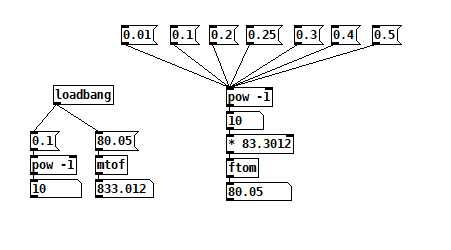period to pitch function.pd
OK, so it looks like it works for all the values you provided except for 0.01, so I'm wondering if sigmund is giving you the wrong answer for that input, or maybe sigmund is latching on to some strong upper harmonic. It's hard to say without seeing the patch.

PS: was there any precalculus in any of that?

• @jameslo As [vline~] is involved then the scheduling could be correct even as the control is at control rate.
Not doing much audio work in Pd I hadn't realised that [sigmund~] exports midi notes rather than frequency.... thanks for spotting and pointing that out....!
It explains the non-linearity..... fm = 2((m−69)/12))*440 where fm is the frequency of the midi note m.
That is..... frequency = 2 (to the power of (m minus 69) divided by 12) times 440.
But the pitch as a frequency relative to time is a simpler calculation..... and remains linear as above.
An upper harmonic is more likely... note 96 (about 2000Hz) is well within range for [sigmund~] which struggles at very low frequencies.
As you say, the array will reveal all....... and the patch especially as it will show whether [vline~] is controlled correctly for scheduling.
I hope the whiskey was a good one.
David.
For @murkrellr ........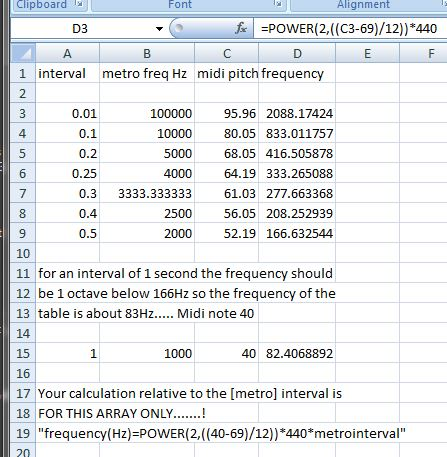But of course if you have an array that reports a different midi note in [sigmund~] for a metro interval of 1 then you can replace the "40" with that value in your calculation.

And....... in this case...... the frequency(Hz) = interval/1000/12.135 (ish).
Taking the reported midi value value for the 0.1 interval matches the series better so.....
Frequency (Hz) = 83.3 / metro value

• Another approach is to design the implementation around the requirement.

You want to be able to produce a specific frequency. So... make frequency the input parameter, rather than metro time interval.

You want to step through array values, with an equal amount of time for each. That suggests a linear ramp, with a given frequency... i.e., phasor~.

phasor~ always goes 0.0 - 1.0. With 12 points, the array indices go 0.0 - 11 -- but here, note that if you say 0.0 - 11.0, then index 0 covers a span of 1, but index 11 covers a span of 0! That doesn't sound right. Instead, define the span for each index as i <= x < (i+1) -- inclusive at the bottom, exclusive at the top. Now the entire range is 0 <= x < 12... leading to the idea of multiplying the phasor by 12 (number of points).

The array indexing should truncate away any fractional part: if (phasor * 12) = 3.546, it should just read 3. Fortunately, this is the normal behavior of tabread~ (not tabread4~).

So we can get a sample-and-hold waveform this way. (The third array is just showing the floor-ed indices, to demonstrate that tabread~ really is truncating rather than rounding.)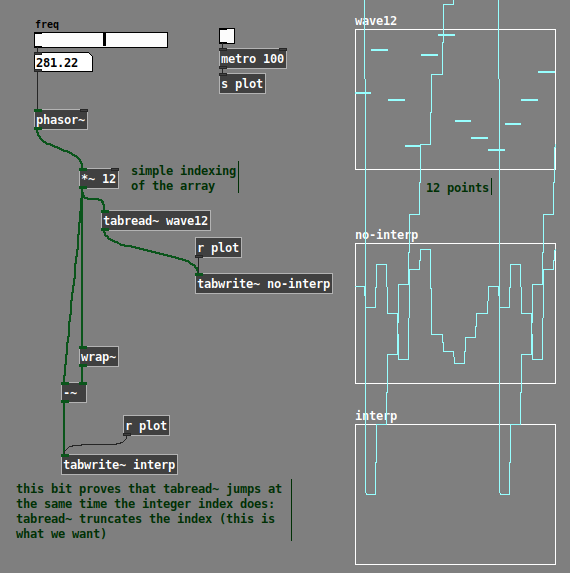All that's left is to add linear interpolation. A standard way to do linear interpolation is:

x = crossfade factor (0.0 - 1.0)
a = value when x = 0
b = value when x = 1

xfade = (b-a) * x + a (this is a reduction of `(a * (1-x)) + (b * x)`).

If i is the index, then a = array "at" i, and b = array "at" i+1 -- but here, b needs to be modulo-wrapped back around, because i+1 could be 12-point-something. Pd vanilla doesn't have [%~] at signal rate, but [expr~] has fmod().

So we can index the two values, subtract, multiply by the crossfade factor (which is phasor * 12 --> wrap~), then add the "array[i]" value back in.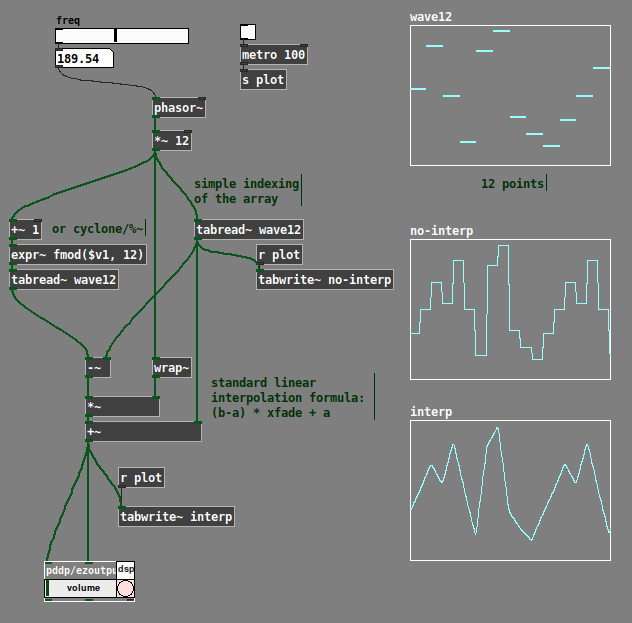23-0408-linear-gendy-2.pd

Now you can do whatever frequency you want, without the awkward conversion. Doesn't matter what that online tutorial said, IMO this fits the stated requirement better.

hjh

• @lacuna said:

It depends on which control objects are involved and what exactly you are doing, but [metro] can be sample accurate:

Ah, thanks for this. Indeed, I had assumed control messages are quantized to control block boundaries. Now I see that the message transmission happens on the block boundaries, but the logical time associated with the message doesn't have to be quantized (and objects like vline~ can use this for sub-block timing).

(But I still think, in this specific case, it looks more straightforward to me to keep it all in the signal domain.)

hjh

•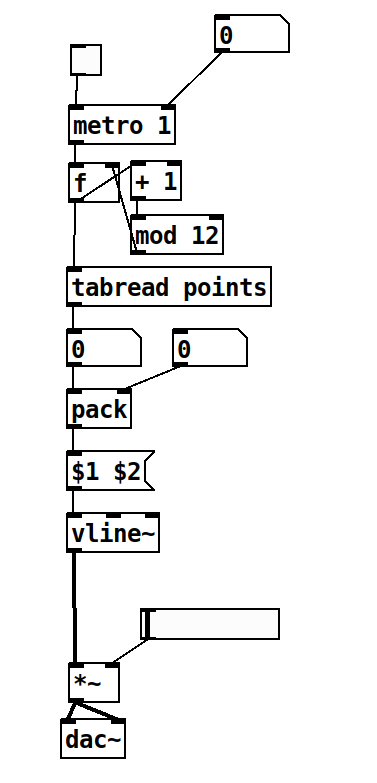here is the patch in question. it is from QCG Interactive Music tutorial lesson #47 here..

I had made this while watching tutorials and went back to play around with it.
in the video he says something like "and ill leave it to you to figure out" in regards to this, and so
I sat out to do that since it seemed interesting... and I got so far as coming up with using sigmund~ to detect the pitches i get as a result of moving the metro speed from 0.01-2.

I am at this point attempting to learn by solving mysteries that arise on my way to building an idea.

thanks to everyone who gave this question some attention! ill be studying this for a while.

• @murkrellr Here is a slightly extended version of the patch, also allowing to randomly vary the time of each segment (which Xenakis described in his book "Formalized Music"). I hope my comments help to clarify the conversion from metro time to pitch: gendy.pd
I have to say I kind of like that dirty sound ...• There is, by the way, a simple explanation, why your first measurement didn’t match the true mathematical relationship between metro time and pitch (and therefore made it pretty much impossible to guess just by looking at the numbers):
Let’s say we have a sample rate of 48000 Hz, which means 48000 samples per second. Then the duration of one sample is 1/48000 s or about 0.02 ms. So if you run the metro at 0.01 ms, one period of the wave would only have about 6 samples! Now it’s obvious that it’s impossible to create line segments between 12 points with just 6 samples, isn’t it?
If you are interested in the effects of sampling more generally, you may want to search for “aliasing” or “foldover”. It’s an important topic in all of DSP, so it might be worth it.

• @manuels said:

Let’s say we have a sample rate of 48000 Hz, which means 48000 samples per second. Then the duration of one sample is 1/48000 s or about 0.02 ms. So if you run the metro at 0.01 ms, one period of the wave would only have about 6 samples! Now it’s obvious that it’s impossible to create line segments between 12 points with just 6 samples, isn’t it?

That's a good catch.

The phasor~ approach that I demo'ed would inherently decimate the given waveform -- plenty of aliasing but the frequency would be correct. However that approach seemed not to be very popular in the thread(though I have to admit it wouldn't handle the random segment time gracefully -- you could modulate the phasor~ frequency but the ramps between points would not be straight lines -- I thought about how to do that but it seems tricky).

hjh

• it is no problem to read from a wavetable in cycles smaller than 1 sample, as long as the wavetable object is able to interpolate.

but running a metro at 0.01 ms might not work for some other reasons,when trying to "find the right math" the most important thing is that you are aware about what you want to find.

for example the OP is talking about "conversion" from "speed" to "pitch" and later he calls it just "this".

in this situation it can help to write down what the different objects and their functionality actually stands for and break down the problem into smaller parts.

for a wavetable oscillator the following is true: if it is played with a rate of 1.0, its pitch will be a product of its length and the system samplerate,

so write down these values and units and see how they fit together with the rest of the patch.

after you figured this out, the next step could be to find the formula to make it cycle at 261Hz when a middle C is coming in under the condition of a variable system samplerate. this now will only be a rule of three job.

unlike others i dont think you need to learn more about generic maths. the trick is rather to fully understand your own patch.

Posts 20 | Views 837
Internal error.

Oops! Looks like something went wrong!